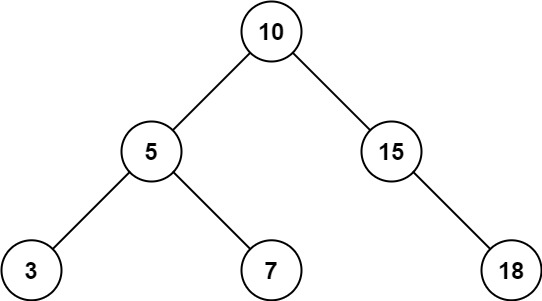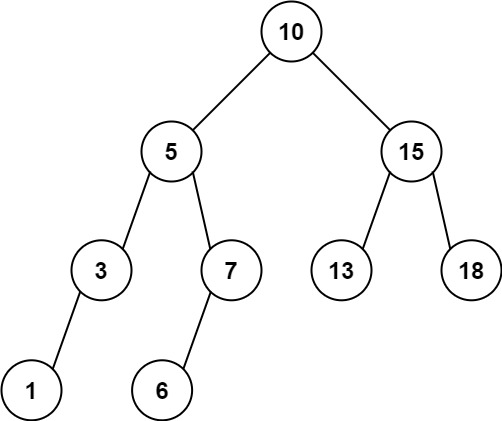# GeetCode Hub

Given the `root` node of a binary search tree and two integers `low` and `high`, return the sum of values of all nodes with a value in the inclusive range `[low, high]`.

Example 1:```Input: root = [10,5,15,3,7,null,18], low = 7, high = 15
Output: 32
Explanation: Nodes 7, 10, and 15 are in the range [7, 15]. 7 + 10 + 15 = 32.
```

Example 2:```Input: root = [10,5,15,3,7,13,18,1,null,6], low = 6, high = 10
Output: 23
Explanation: Nodes 6, 7, and 10 are in the range [6, 10]. 6 + 7 + 10 = 23.
```

Constraints:

• The number of nodes in the tree is in the range `[1, 2 * 104]`.
• `1 <= Node.val <= 105`
• `1 <= low <= high <= 105`
• All `Node.val` are unique.

/** * Definition for a binary tree node. * public class TreeNode { * int val; * TreeNode left; * TreeNode right; * TreeNode() {} * TreeNode(int val) { this.val = val; } * TreeNode(int val, TreeNode left, TreeNode right) { * this.val = val; * this.left = left; * this.right = right; * } * } */ class Solution { public int rangeSumBST(TreeNode root, int low, int high) { } }